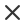ФильтрТип носителя
Тип лицензии
Ориентация
Style
Исключать

# Physics Набор Векторов и Иллюстраций

Physical formulas and phenomenon hand drawn illustration science board with math physics education at school vector illustrationMath education vector pattern with handwritten formulas tasks plots calculations and geometrical figures vector illustrationYoung woman sitting on the floor and solving math problem with black board female person dressed in casual clothes study at home vector flat color illustrationBlackboard inscribed with scientific formulas and calculations in physics and mathematics can illustrate scientific topics tied to quantum mechanics relativity theory and any scientific calculations vector illustrationSeamless pattern on the theme of the subject of geometry formulas and charts of theorems a bright outline on a blue backgroundMathematical vector seamless pattern with plots formulas geometrical figures and calculations you can use any color of backgroundPhysical formulas graphics and scientific calculations back to school science lab objects doodle vintage style sketches on aged paper background vintage hand drawn illustration3d connection structure futuristic technology style low poly element for design vector illustration for science chemistry or educationMath formula mathematics calculus on school blackboard algebra and geometry science chalk pattern vector education concept scientific analysis number calculation complex knowledgeAbstract futuristic network shapes high tech hud background connecting lines and dots polygonal linear texture world globe on blue global network connections geometric design dig data conceptMathematical vector scientific technical seamless pattern with handwritten formulas calculations equations on a green backgroundAi cyber mind and digital technology big data analysis machine learning and artificial intelligence concept scientific innovations ai development business presentation of human brain analysisPhysics chemistry biology and astronomy science doodle handwriting theory and tool icon pouring from lab beaker bottle in blackboard background paper used for school education and document decoration create by vectorLightning collision red and blue background versus banner powerful colored lightnings and the flash from the collision confrontation concept competition vs match game versus battle vectorSeamless pattern on the theme of the subject of geometry formulas and charts of theorems a bright outline on a dark backgroundMath theory mathematics calculus on class chalkboard algebra and geometry science handwritten formulas vector education concept formula and theory on blackboard science study illustrationCrimean bridge after destruction with smoke on a blue background stock vector illustration of destroyed infrastructureBig artificial brain or supercomputer with ai via wires connected with human brain deep learning of neural networks artificial intelligence concept sci fi illustration of tech genius or super humanPhysical vector seamless pattern with formulas equations and figures handwritten on a blackboard seamless designMath linear mathematics education circle background with geometrical plots formulas and calculations design concept vector illustrationMath education vector pattern with handwritten formulas tasks plots calculations and geometrical figures vector illustrationAbstract artificial intelligence dna technology science and social concept futuristic connection structure with cell backgroundMinimalism multi colored glass hi tech abstract background design glowing neon technology rgb double glazed windows layout cover vector illustrationPhysical vector seamless pattern with formulas equations and figures handwritten on a blackboard seamless designPhysical formulas graphics and scientific calculations on chalkboard vintage hand drawn illustration laboratory seamless patternNotes on exercises physics formulas and equations uniform rectilinear motion statics electromagnetism electrical circuits friction force energy angular velocity on a grid sheetMath linear mathematics education circle background with geometrical plots formulas and calculations design concept vector illustrationVector abstract golden luxury pattern lines background line arts wallpaper wavy art deco texture for print fabric packaging design sea ocean landscape art in japanese style3d molecular structure with spherical particles futuristic technology style vector illustration for science chemistry or educationQuantum processing deep learning artificial intelligence future new technology for business or science presentation vector backgroundEndless mathematical texture with geometrical figures plots and equations handwritten with chalk on a grey blackboard mathematical vector seamless patternSocial network media global people communication and information sharing 3d web isometric illustration infographic concept vector network connection business